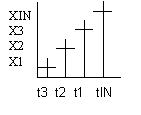Product : ISaGRAF V3

Date    : 29-March-1999

File    : Derivate.htm

Subject : Derivate algorithm

Keywords: Derivate - standard function block - C function block

____________________________________________________________________

Here is the algorithm of the standard C function block Derivate:

Arguments:

RUN BOO mode: TRUE=normal / FALSE=reset

XIN REAL input: any real analog value

CYCLE TMR sampling period

XOUT REAL differentiated output

Description:

Differentiation of a real value.

Algorithm:

If the "CYCLE" parameter value is less than the cycle timing of the ISaGRAF application,

the sampling period is the cycle timing of the application.

Here are the local variable manipulated by this block:

DER_INIT_DONE Bool

DER_X1 Real

DER_X2 Real

DER_X3 Real

IF (NOT DER_INIT_DONE) THEN /* only first time */

DER_X1 = XIN;

DER_X2 = XIN;

DER_X3 = XIN;

DER_INIT_DONE = 1;

END_IF;

IF (RUN) THEN

/* delay is the current cycle time */

IF ((delay) AND (delay > CYCLE)) THEN

XOUT = (3.0 * (XIN - DER_X3) + DER_X1 - DER_X2) / (TIME_TO_REAL)delay;

DER_X3 = DER_X2;

DER_X2 = DER_X1;

DER_X1 = XIN;

END_IF;

END_IF

Notes:

If you look at the Xout calculation, and following this diagramWe see that we can consider that

XOUT = (3.0 * (XIN - DER_X3) + DER_X1 - DER_X2) / (TIME_TO_REAL)delay;

is approximatively

3*(3*DelatX) + DeltaX / cycleTime

so 10* DeltaX / cycleTime

so the value is 10 times the value of the slope. (unit 1ms/10)

____________________________________________________________________